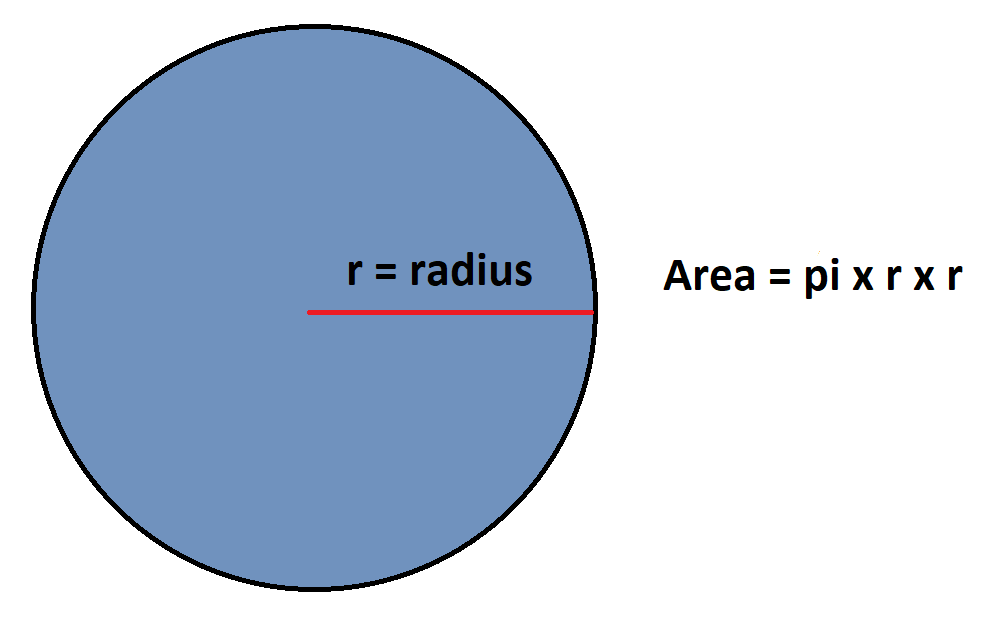# Perimeter and Area of a Circle

Go back to  'Mensuration and Measurement'

## Introduction to Perimeter and Area of a Circle

Imagine a circular slice of pizza. If you know the radius (or diameter) of the pizza, you can easily find its perimeter and area. This was just one example, but there are several situations which need you to know the area and perimeter of a given circle, and we will discuss those ways today.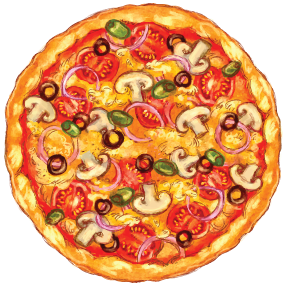## What is pi ?

Before jumping into the technicalities, let’s familiarize ourselves with the diameter and the perimeter or circumference of a circle. To visualise the circumference, think of the crust of a Pizza, well, that’s essentially what it is! The length of the outer edge of any circle.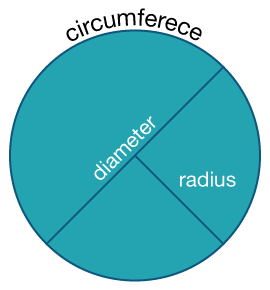Here’s where things get really interesting. What if you were told that if you divide the circumference of ANY circle with its diameter, you will ALWAYS end up with the SAME number, 3.1415…

The use of ellipses is deliberate! This particular ratio is always the same regardless of the circle in question! Yes, it is mind boggling, which is why this number occupies such a crucial role in all of the mathematics related to the circle. This ratio is called Pi.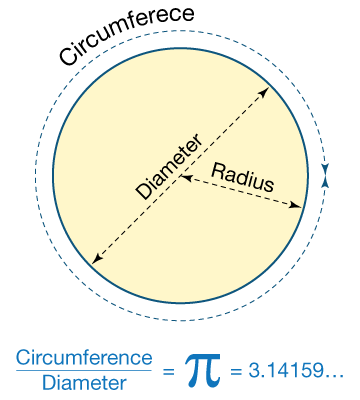Regarding the number, pi is a non-repeating, non-terminating entity which is best approximated to the fraction $${22 \over 7}$$. The decimal representation up to 10 places is 3.1428571429

## How is it important?

### The perimeter of a circle

Like we understood for closed polygons bounded by straight lines, the perimeter of a circle is the length of the boundary enclosing that circular space. If you imagine a perfectly circular field with a white fence all around it, then the white fence can be said to represent the perimeter of the circular field. If you think of a pizza, then the outer crust of the pizza can be said to be its perimeter. For a circular space of radius $${r}$$, the perimeter is calculated as

$${\text{Perimeter}} = 2 \times \pi \times r = 2\pi r$$

We do know that the radius of a circle is half its diameter, and if $${\text{D}}$$ represents the diameter of the circle, then $${\text{D} = 2r}$$. If we replace $${2r}$$ of the perimeter formula by $${\text{D}}$$, then,

\begin{align}{\text{Perimeter}} = \pi D\end{align}

If you have purchased a 14-inch pizza, you now know how to calculate the length of the outer boundary of the pizza.

\begin{align}{\text{Perimeter}} = \pi D = \frac{{22}}{7} \times 14 = 44{\text{ inches}}\end{align}

Let’s have a look at the circumference of the circle visually, so that the concept can be more easily internalized.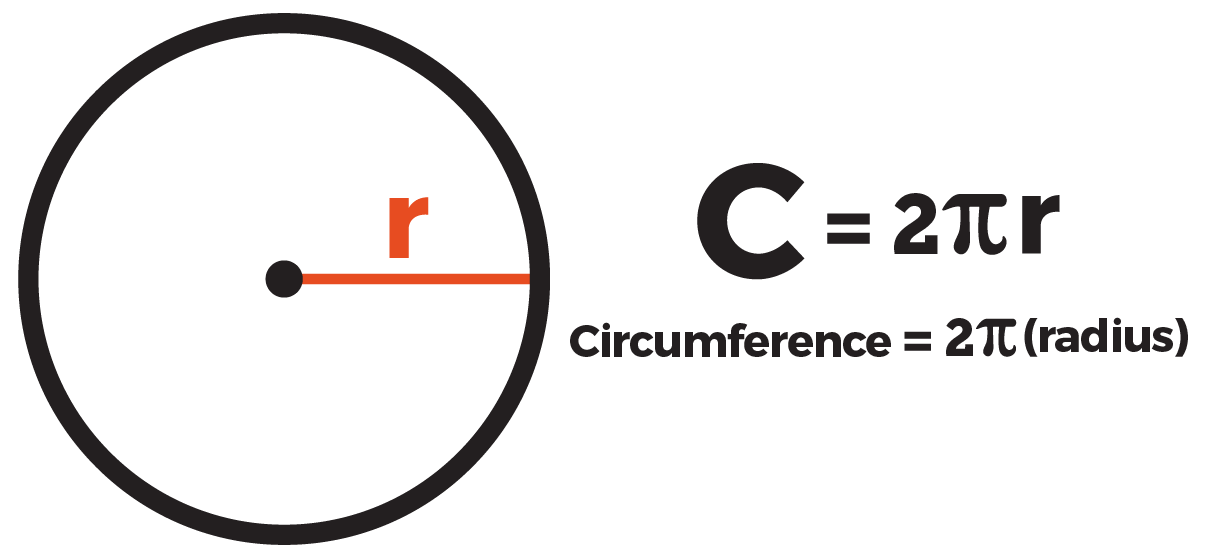### Area of a Circle

We spoke some time back about the length of the fencing required to close a circularly shaped lawn completely. But what if you need to find out how much space the lawn covers to that you can find out the amount to be paid to a gardener who charges by the area he has mown? Or if you want to find out the space covered by the circular pizza; you will need to find out its area. The area of a circle again is a function of π and its radius, but the radius is considered in two dimensions.

$${\text{Area of a circle = }}$$$${πr^2}$$

Since the Diameter D of a circle is twice the radius, therefore we can also replace the radius r by $${D \over 2}$$ in the above formula and get

$${\text{Area of a circle = }}$$$${πD^2 \over 4}$$

When we ask the question, “How much space does the pizza enclose?” What is implied may be visualized using the image below. The space enclosed is represented by the shaded (blue) area.